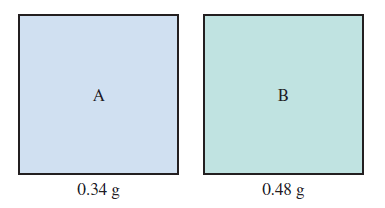# Problem: Consider two gases, A and B, each in a 1.0-L container with both gases at the same temperature and pressure. The mass of gas A in the container is 0.34 g and the mass of gas B in the container is 0.48 g.c. Which gas sample has the fastest average velocity? Explain.

###### FREE Expert Solution
100% (467 ratings)
###### Problem Details

Consider two gases, A and B, each in a 1.0-L container with both gases at the same temperature and pressure. The mass of gas A in the container is 0.34 g and the mass of gas B in the container is 0.48 g.c. Which gas sample has the fastest average velocity? Explain.

Frequently Asked Questions

What scientific concept do you need to know in order to solve this problem?

Our tutors have indicated that to solve this problem you will need to apply the Kinetic Energy of Gases concept. You can view video lessons to learn Kinetic Energy of Gases. Or if you need more Kinetic Energy of Gases practice, you can also practice Kinetic Energy of Gases practice problems.

What textbook is this problem found in?

Our data indicates that this problem or a close variation was asked in Chemistry: An Atoms First Approach - Zumdahl Atoms 1st 2nd Edition. You can also practice Chemistry: An Atoms First Approach - Zumdahl Atoms 1st 2nd Edition practice problems.